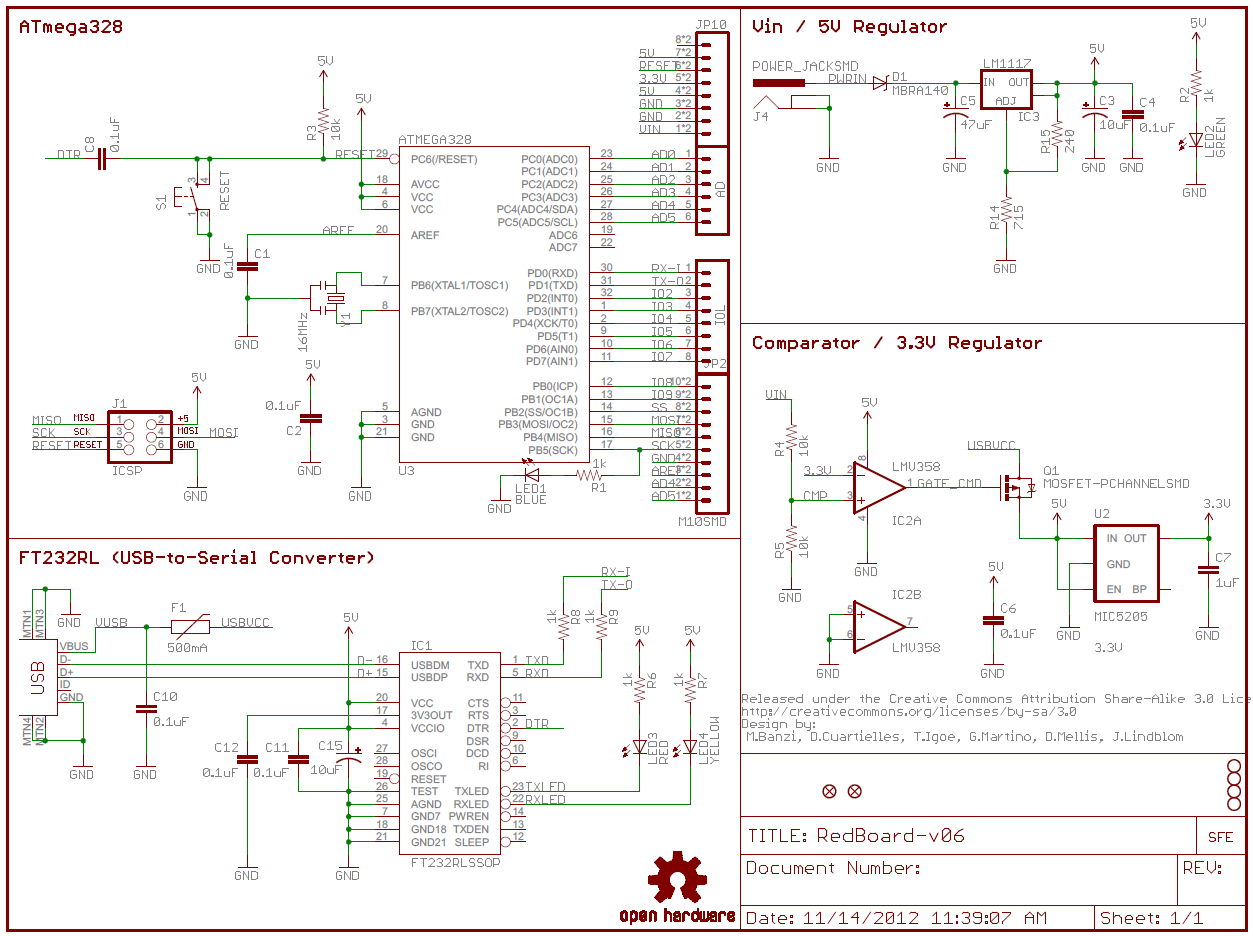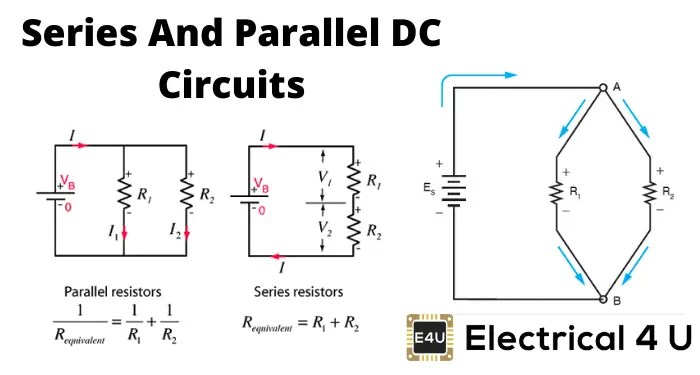# Circuit Diagram Explained: A Comprehensive Guide

Circuit diagrams are a crucial part of any electrical engineering project. They provide a visual representation of how electrical circuits are wired and connected, allowing engineers to quickly troubleshoot issues and improve the design of their projects. Understanding circuit diagrams can help anyone interested in electrical engineering or electronics to better understand and develop their projects. In this comprehensive guide, we will explain what circuit diagrams are, how they are created, and how they can be used.

## What is a Circuit Diagram?

A circuit diagram is a visual representation of an electrical circuit. It shows the components of the circuit as well as their connections. It also shows the wiring of the circuit and the power sources. Circuit diagrams are used to troubleshoot problems, design new circuits, and understand existing circuits. Circuit diagrams can also be used to test circuits, as they provide a clear view of all the components and connections.

## How Are Circuit Diagrams Created?

Circuit diagrams are usually created using special software. This software allows the user to draw out the circuit, add components, and connect them together. The user can then add labels to the components and create a neat and organized diagram. This diagram can then be used to troubleshoot problems, test the circuit, and compare it to other circuits.

## Types of Circuit Diagrams

There are several types of circuit diagrams, each with its own purpose. The most common types of diagrams are:

• Schematic diagrams – These diagrams show the connections between components in a schematic form. Schematic diagrams are used to troubleshoot problems, design new circuits, and understand existing circuits.

• Wiring diagrams – These diagrams show the connections between components in a detailed form. Wiring diagrams are used to understand existing circuits, and to install, modify, and repair circuits.

• Block diagrams – These diagrams show the components of a system in a simplified form. Block diagrams are used to understand complex systems, such as computers and communications systems.

## Using Circuit Diagrams

Circuit diagrams can be used in a variety of ways. They can be used to troubleshoot problems in existing circuits, or to design and test new circuits. They can also be used to understand existing circuits, install and modify circuits, and repair circuits. Circuit diagrams can also be used to compare different circuits, and to understand complex systems, such as computers and communications systems.

## Conclusion

Circuit diagrams are an essential tool for any electrical engineer. They provide a visual representation of an electrical circuit, showing all its components and connections. Circuit diagrams can be used to troubleshoot problems, design new circuits, and understand existing circuits. They can also be used to install, modify, and repair circuits, as well as compare different circuits. Understanding circuit diagrams can help anyone interested in electrical engineering or electronics to better understand and develop their projects.How To Read A Schematic Learn Sparkfun ComCircuit Diagram How To Read And Understand Any SchematicHow To Read A Schematic Learn Sparkfun ComExamples Of Electronic Schematic DiagramsWhat Is A Schematic And Circuit Diagram QuoraWiring Diagram Everything You Need To Know AboutCircuit Diagram Of The Model Diffe Sections Schematic ScientificHow To Read A Schematic Learn Sparkfun ComCircuit Diagram And Its Components Explanation With SymbolsCircuit Diagram To Measure The Topological Uhlmann Phase E G With ScientificWhat Is Relay Switch Circuit Diagram And Working Principle EtechnogElectric Circuit Diagram Images Browse 17 972 Stock Photos Vectors And AdobeElectrical Symbols CircuitsHow To Read A Schematic Learn Sparkfun ComXkcd Circuit DiagramEdge Computing Circuit Diagram Explained By It Expert Stock Photo Beebright 173347088How To Read A Circuit Diagram Archives UpmationSimple Inverter Circuit Diagram Apps Bei Google PlaySeries And Parallel Dc Circuits Explained Examples Included Electrical4u